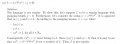# Proving a^ib^ja^(i.j) | i, j > =0; using Pumping Lemma

#### zulfi100

Joined Jun 7, 2012
617
Hi,
I have to prove:
(a^i) (b^j) (a^(i.j) ) | i, j > =0; non regular using pumpimg Lemma. I have to suppose a string.

Let w = (a^m) . (b^m) . (a^(m.m))

I have to find xy in this string such that |xy| >= m
(a^m) = |xy|
(b^m) = |xy|
(a^(m.m)) = |xy| + |xy|

Now xyz = (a^m) ...Problem?

What would be 'z' here? Some body please guide me.

Zulfi.

#### WBahn

Joined Mar 31, 2012
24,854
Is (i.j) in your language definition i multiplied by j? That's what I will assume.

Why do you have to find xy such that |xy| >= m?

I'm assuming that m is a pumping length for this language?

If you have defined what x and y are, the z has no choice but to be everything else in the original string the follows the prefix xy.

Remember what the pumping lemma for regular languages says -- and what it does not say. You have to PROVE that there is NO WAY to partition the chosen string so that it is pumpable. You don't get to cherry pick.

#### zulfi100

Joined Jun 7, 2012
617
Is (i.j) in your language definition i multiplied by j? That's what I will assume.
Yes.
Why do you have to find xy such that |xy| >= m?
Sorry it was a mistake, I have to find |xy| <=m. It is a necessary condition.
Next step is to find 'y' such |y|>=1.

If you have defined what x and y are, the z has no choice but to be everything else in the original string the follows the prefix xy.
I have found |xy|, but I have to find 'y'.

If (a^m) = |xy| then 'y' can be the last 'a'. Am i right?
Now xyz = (a^m) . (b^m) . (a^(m.m))
z= (b^m) . (a^(m.m))

Now from pumping Lemma xy^iz belongs to L
Consequently xy^0z must belong to L. Since |xy| <=m & |y| > 0 so
x.y^(0). z= (a^(m-|y|)). (b^m).(a^(m.m)) belongs to L but its a contradiction because the first two terms i.e. (a^m) & (b^m) have equal powers. So L is not regular.

Is the above correct?
I am attaching the solution attached.

Zulfi.#### zulfi100

Joined Jun 7, 2012
617
Hi,
I tried to prove another expression as non-regular using pumping Lemma.
L={(01)^m. 2^m | m>=0}
Suppose L is regular. Since L is regular and infinite PL holds. Let m > 0 be the integer in the PL. Pick a string w such that w belongs to L.
We pick w = (01)^m 2^m,
|xy| <= m , |y| >=1, x.y^k .z belongs L

(01)^m = |xy|
y = last 01 of xy
& z = 2^m
Now x.y^i . z belongs to L where i = 0,1, 2, ..
Consequently xy^2z belongs to L. |xy| <= m & |y| > 0
So x.y^2 .z = (01)^(m+1) . 2^m. This shows that x.y^2.z does not belong to L. This is a contradiction. So L is not regular.

Kindly check this one also.

Zulfi.

#### WBahn

Joined Mar 31, 2012
24,854
Yes.

Sorry it was a mistake, I have to find |xy| <=m. It is a necessary condition.
Next step is to find 'y' such |y|>=1.

I have found |xy|, but I have to find 'y'.

If (a^m) = |xy| then 'y' can be the last 'a'. Am i right?
Now xyz = (a^m) . (b^m) . (a^(m.m))
z= (b^m) . (a^(m.m))
This is cherry picking.

This is ONE of the many possible ways to partition the string, and so if THIS partitioning doesn't satisfy the Pumping Lemma, ALL you have accomplished is shown that THIS ONE partitioning doesn't work.

There are many, many other ways to partition the string. What if 'y' is the last two a's? What if x is the empty string in y is the first 'a'? What if x is the first 3 a's and y is next 4 a's?

You have to show that NONE of those partitionings, or ANY OTHER way of partitioning the string, results in a pumpable partitioning.